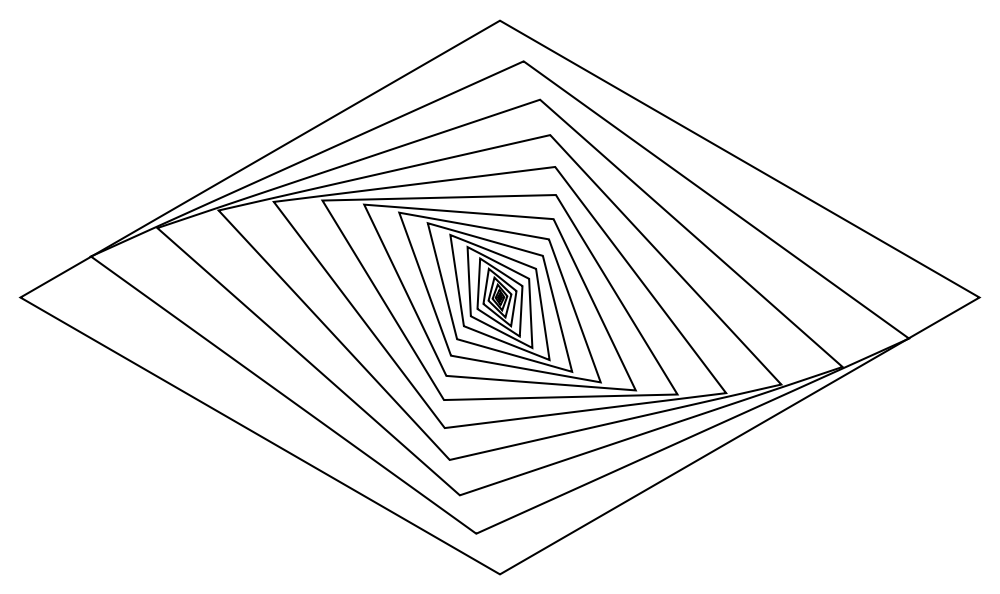#Function Repository Resource:

# Rhombus

Produce a rhombus for use in graphics

Contributed by: Sander Huisman
 ResourceFunction["Rhombus"][] returns an axis-aligned golden rhombus centered around the origin with side length 1. ResourceFunction["Rhombus"][{x,y}] returns an axis-aligned golden rhombus centered around {x,y} with side length 1. ResourceFunction["Rhombus"][{x,y},a] returns an axis-aligned golden rhombus centered around {x,y} with side length a. ResourceFunction["Rhombus"][{x,y},a,θ] returns an axis-aligned rhombus centered around {x,y} with side length a and half-angle θ. ResourceFunction["Rhombus"][{x,y},a,θ,ϕ] returns a rhombus centered around {x,y} with side length a and half-angle θ and tilted at an angle ϕ. ResourceFunction["Rhombus"][{x,y},{p,q}] returns an axis-aligned rhombus centered around {x,y} with semi-axes lengths p and q. ResourceFunction["Rhombus"][{x,y},{p,q},ϕ] returns a rhombus centered around {x,y} with semi-axis lengths p and q and tilted at an angle ϕ.

## Details and Options

The parameters x, y, a, p, q, θ and ϕ are defined as follows: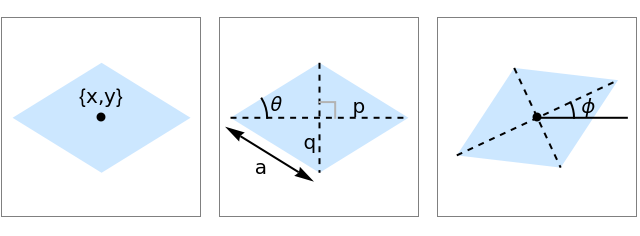## Examples

### Basic Examples

Generate a golden rhombus with unit side lengths centered around the origin:

 In:=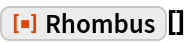Out=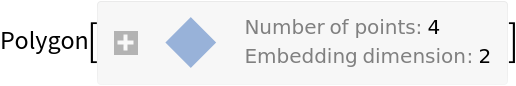Generate a lozenge with unit side lengths centered around the origin:

 In:=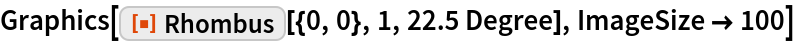Out=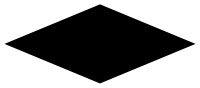### Scope

Create a rhombus defined by its axis lengths:

 In:=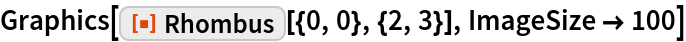Out=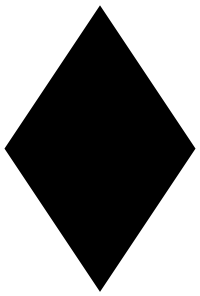Define a rhombus by a side length and an angle:

 In:=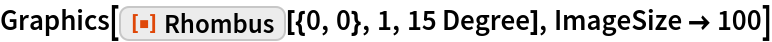Out=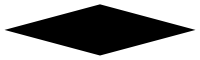Create a rhombus defined by its axis lengths and rotate it 35° counterclockwise:

 In:=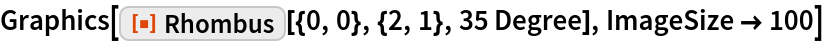Out=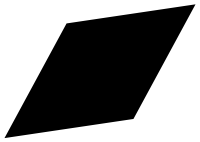### Applications

Use rhombuses as error bars:

 In:=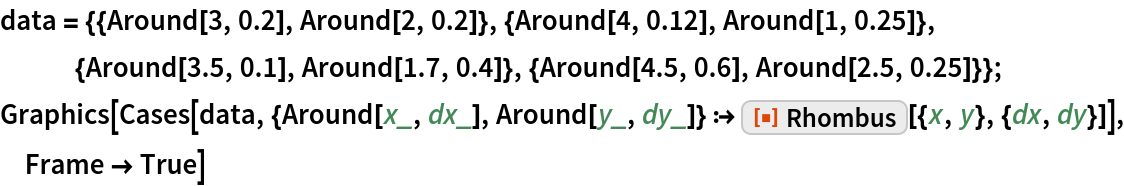Out=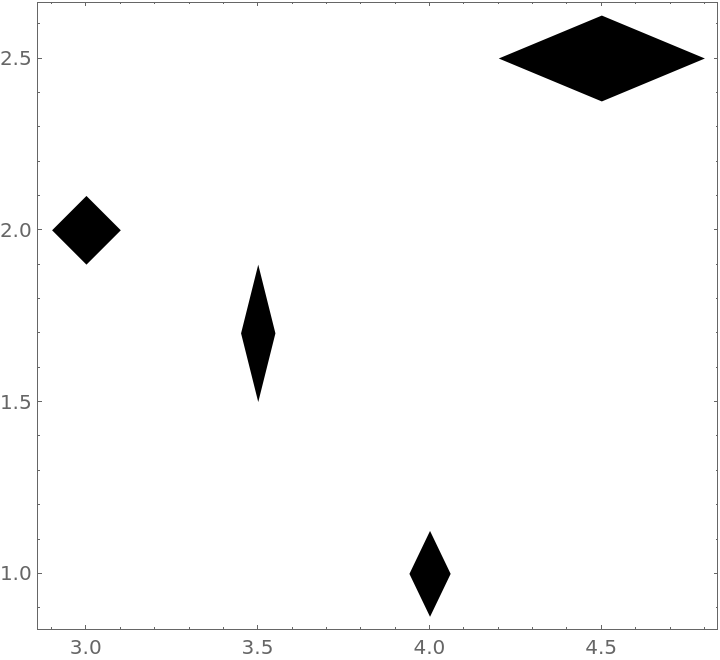### Possible Issues

With an angle of 0 or 180 degrees, the polygon will have no area (a degenerate polygon):

 In:=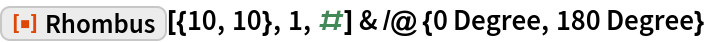Out=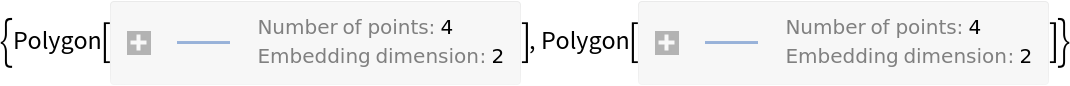### Neat Examples

Create a visually appealing collection of rhombuses:

 In:=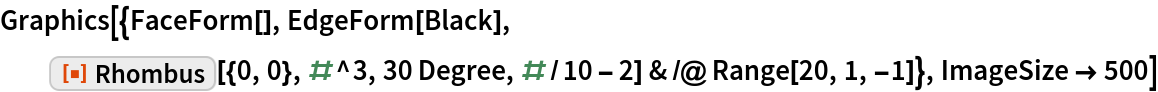Out=